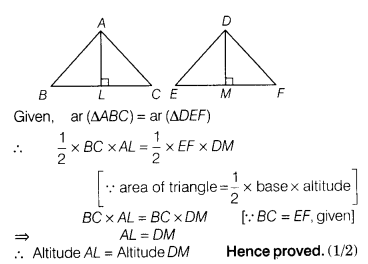# If two triangles have equal area and one side of the one triangle is equal to one side

If two triangles have equal area and one side of the one triangle is equal to one side of the other triangle, then prove that their corresponding altitudes are equal.

Let, ABC and DEF are two triangles such that ar (∆ABC) = ar (∆DEF) and BC = EF

Also, let AL and DM are altitudes of ∆ABC and ∆DEF, respectively.

To prove Altitude AL = Altitude DM